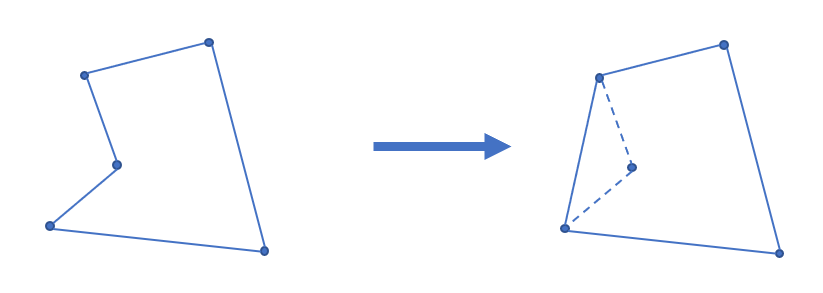# 凸包

## 二维凸包

### 凸包### 凸包的求法

#### Andrew 算法求凸包

  1 2 3 4 5 6 7 8 9 10 11 12 13 14 15 16 17 18 19 20 21 22 23 24 25 26 // C++ Version // stk[] 是整型，存的是下标 // p[] 存储向量或点 tp = 0; // 初始化栈 std::sort(p + 1, p + 1 + n); // 对点进行排序 stk[++tp] = 1; // 栈内添加第一个元素，且不更新 used，使得 1 在最后封闭凸包时也对单调栈更新 for (int i = 2; i <= n; ++i) { while (tp >= 2 // 下一行 * 操作符被重载为叉积 && (p[stk[tp]] - p[stk[tp - 1]]) * (p[i] - p[stk[tp]]) <= 0) used[stk[tp--]] = 0; used[i] = 1; // used 表示在凸壳上 stk[++tp] = i; } int tmp = tp; // tmp 表示下凸壳大小 for (int i = n - 1; i > 0; --i) if (!used[i]) { // ↓求上凸壳时不影响下凸壳 while (tp > tmp && (p[stk[tp]] - p[stk[tp - 1]]) * (p[i] - p[stk[tp]]) <= 0) used[stk[tp--]] = 0; used[i] = 1; stk[++tp] = i; } for (int i = 1; i <= tp; ++i) // 复制到新数组中去 h[i] = p[stk[i]]; int ans = tp - 1; 
  1 2 3 4 5 6 7 8 9 10 11 12 13 14 15 16 17 18 19 20 21 22 23 24 25 26 27 28 29 # Python Version stk = [] # 是整型，存的是下标 p = [] # 存储向量或点 tp = 0 # 初始化栈 p.sort() # 对点进行排序 stk[tp] = 1 tp = tp + 1 # 栈内添加第一个元素，且不更新 used，使得 1 在最后封闭凸包时也对单调栈更新 for i in range(2, n + 1): while tp >= 2 and (p[stk[tp]] - p[stk[tp - 1]]) * (p[i] - p[stk[tp]]) <= 0: # 下一行 * 操作符被重载为叉积 used[stk[tp]] = 0 tp = tp - 1 used[i] = 1 # used 表示在凸壳上 stk[tp] = i tp = tp + 1 tmp = tp # tmp 表示下凸壳大小 for i in range(n - 1, 0, -1): if used[i] == False: # ↓求上凸壳时不影响下凸壳 while tp > tmp and (p[stk[tp]] - p[stk[tp - 1]]) * (p[i] - p[stk[tp]]) <= 0: used[stk[tp]] = 0 tp = tp - 1 used[i] = 1 stk[tp] = i tp = tp + 1 for i in range(1, tp + 1): h[i] = p[stk[i]] ans = tp - 1 

## 三维凸包

### 基础知识

• 首先对其微小扰动，避免出现四点共面的情况。
• 对于一个已知凸包，新增一个点 ，将 视作一个点光源，向凸包做射线，可以知道，光线的可见面和不可见面一定是由若干条棱隔开的。
• 将光的可见面删去，并新增由其分割棱与 构成的平面。 重复此过程即可，由 Pick 定理、欧拉公式（在凸多面体中，其顶点 、边数 及面数 满足 ）和圆的反演，复杂度 1

### 模板题

P4724【模板】三维凸包

  1 2 3 4 5 6 7 8 9 10 11 12 13 14 15 16 17 18 19 20 21 22 23 24 25 26 27 28 29 30 31 32 33 34 35 36 37 38 39 40 41 42 43 44 45 46 47 48 49 50 51 52 53 54 55 56 57 58 59 60 61 62 63 64 65 66 67 68 69 70 71 72 73 74 75 76 77 78 79 80 81 #include #include #include #include using namespace std; const int N = 2010; const double eps = 1e-9; int n, cnt, vis[N][N]; double ans; double Rand() { return rand() / (double)RAND_MAX; } double reps() { return (Rand() - 0.5) * eps; } struct Node { double x, y, z; void shake() { x += reps(); y += reps(); z += reps(); } double len() { return sqrt(x * x + y * y + z * z); } Node operator-(Node A) { return {x - A.x, y - A.y, z - A.z}; } Node operator*(Node A) { return {y * A.z - z * A.y, z * A.x - x * A.z, x * A.y - y * A.x}; } double operator&(Node A) { return x * A.x + y * A.y + z * A.z; } } A[N]; struct Face { int v; Node Normal() { return (A[v] - A[v]) * (A[v] - A[v]); } double area() { return Normal().len() / 2.0; } } f[N], C[N]; int see(Face a, Node b) { return ((b - A[a.v]) & a.Normal()) > 0; } void Convex_3D() { f[++cnt] = {1, 2, 3}; f[++cnt] = {3, 2, 1}; for (int i = 4, cc = 0; i <= n; i++) { for (int j = 1, v; j <= cnt; j++) { if (!(v = see(f[j], A[i]))) C[++cc] = f[j]; for (int k = 0; k < 3; k++) vis[f[j].v[k]][f[j].v[(k + 1) % 3]] = v; } for (int j = 1; j <= cnt; j++) for (int k = 0; k < 3; k++) { int x = f[j].v[k], y = f[j].v[(k + 1) % 3]; if (vis[x][y] && !vis[y][x]) C[++cc] = {x, y, i}; } for (int j = 1; j <= cc; j++) f[j] = C[j]; cnt = cc; cc = 0; } } int main() { cin >> n; for (int i = 1; i <= n; i++) cin >> A[i].x >> A[i].y >> A[i].z, A[i].shake(); Convex_3D(); for (int i = 1; i <= cnt; i++) ans += f[i].area(); printf("%.3f\n", ans); return 0; } 

### 例题

UVA11626 Convex Hull

「USACO5.1」圈奶牛 Fencing the Cows

POJ1873 The Fortified Forest

POJ1113 Wall

「SHOI2012」信用卡凸包